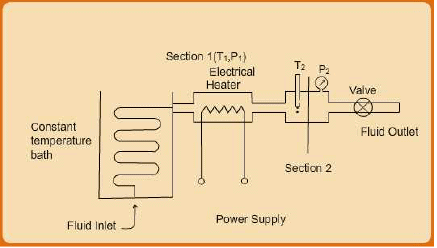Courses

# Measurement of Enthalpy and Internal Energy using Flow Calorimeter Civil Engineering (CE) Notes | EduRev

## Civil Engineering (CE) : Measurement of Enthalpy and Internal Energy using Flow Calorimeter Civil Engineering (CE) Notes | EduRev

The document Measurement of Enthalpy and Internal Energy using Flow Calorimeter Civil Engineering (CE) Notes | EduRev is a part of the Civil Engineering (CE) Course Thermodynamics.
All you need of Civil Engineering (CE) at this link: Civil Engineering (CE)

Measurement of Enthalpy and Internal Energy using Flow Calorimeter

The use of the first law for open or closed systems necessitates the experimental determination (or, estimation from thermodynamic relations) of internal energy and enthalpy, both being state properties. The flow calorimeter (fig. 3.8), readily allows measurement of enthalpy, which in turn can be used to compute the internal energy at the same conditions of (say) temperature and pressure. The fluid whose properties are to be measured is pumped through a constant temperature bath so that it attains a desired temperature (say T1) prior to entry into the vessel provided with an electric heater. The corresponding pressure (P1) may also be recorded. Heat is next provided to the passing fluid at a pre-determined and fixed rate over a period of time until the temperature and pressure at the exit of the vessel attains steady values (say, T2 , & P).  At such a condition the calorimeter is under steady state, hence eqn. 3.41 may be applied to the heating vessel. Since there is no work transfer of any kind, the resultant energy balance yields:

ΔH = Q                   ...(3.40)
Or: H2 – H1 = Q

So: H2 = H1 + Q                   ...(3.41)Clearly, if we chose the enthalpy H1 to be a datum state and arbitrarily assign it a zero value, then:

H2 = Q                   ...(3.42)

he last equation then allows one to uniquely determine the value of H2 at any condition achieved at state 2 by applying a known quantity of heat through the electric heating system. The internal energy at the same state can next be determined using the relation:

U = H – PV

Further we can measure the density (in terms of mass or mole) at the same state, and one may rewrite the last equation as:

U = H – P/ρ         ..(3.43)

Thus using the above relations, U and H may be obtained experimentally at any P, T (and/or V) for any substance. The steam tables discussed at the concluding portion of section 2.1 constitutes such a tabulation of values of standard thermodynamic properties (saturation vapour pressure, internal energy, enthalpy (and entropy) of water obtained empirically over a wide range temperature and pressure.

Offer running on EduRev: Apply code STAYHOME200 to get INR 200 off on our premium plan EduRev Infinity!

## Thermodynamics

56 videos|92 docs|33 tests

,

,

,

,

,

,

,

,

,

,

,

,

,

,

,

,

,

,

,

,

,

;